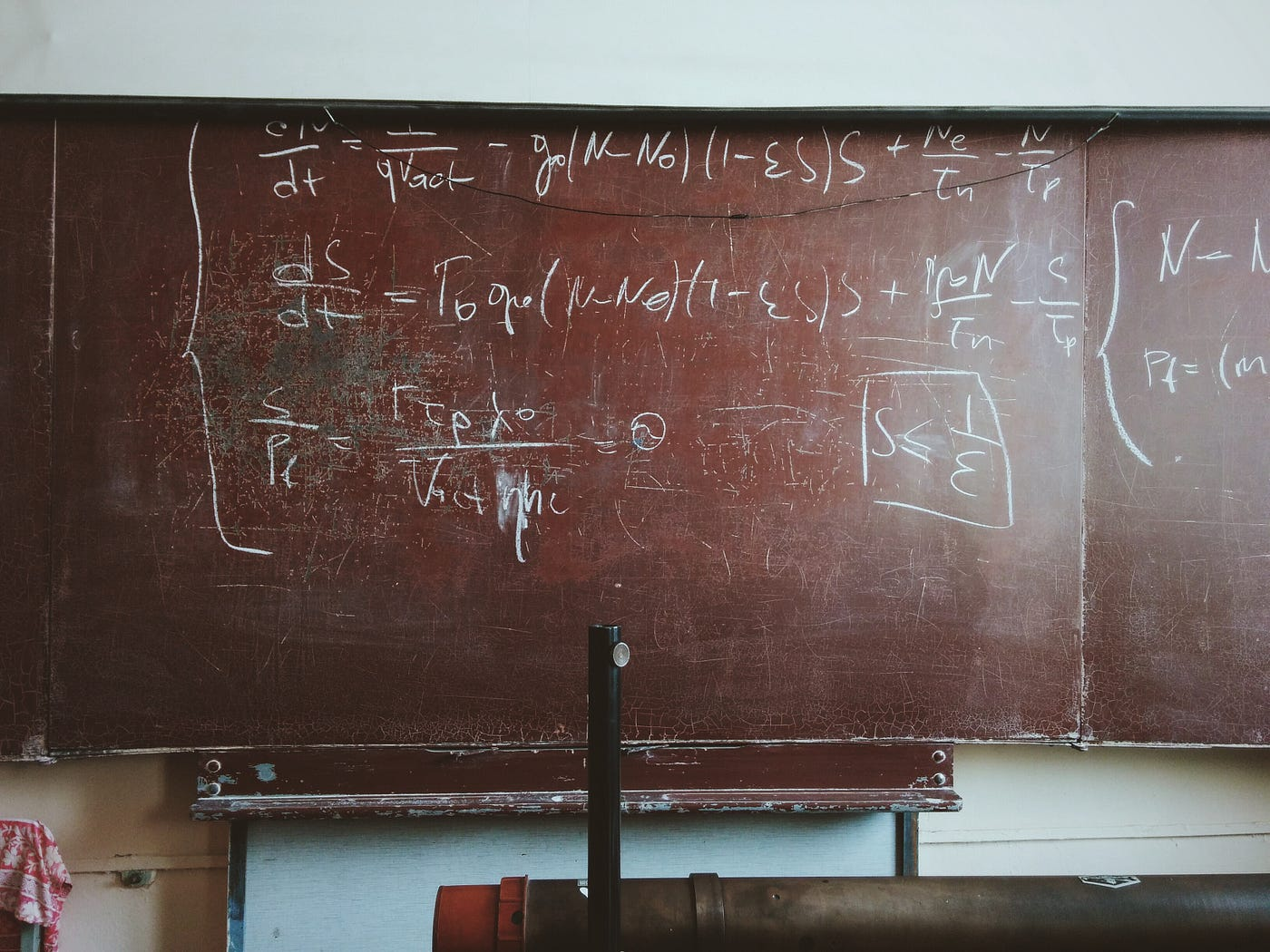# Maths Club: From Circles to Approaching SquaresPhoto by Roman Mager on Unsplash

Monday was the final meeting of maths club before Christmas, we explored the graphs of equations of circles, going into the realm of analyzing equations x⁴ +y⁴ = 1 and x³ +y³ = 1.

# The Unit Circle

The unit circle is a circle of unit radius centered at the origin in the Cartesian plane. It is represented by the equation x² + y² = 1. Knowledge of…

--

--